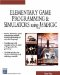# Appendix B: JAMAGIC Language Reference

In this appendix, we present some of the more useful commands in Jamagic and explain how to use them. Please see the Help section of the software for a full listing of the commands.

## Abs

This command returns the absolute value of a number. Here is its syntax:

` x = abs(y) `

### Parameters

 x The absolute value of y

### Notes

The absolute value of a number is a very simple concept; it is the same number with the opposite sign. For instance, the absolute value of “5 is +5.

This function can be useful when you are trying to display distances between objects. For example, if you are interested in displaying the x distance between two objects, you might subtract the x coordinate of one from the x coordinate of the other. However, at times, this might result in negative numbers , which might confuse the game user . By using the absolute value function, you can ensure that a positive value is printed to the screen.

### Sample Code

The following code prints the absolute value of the distance between the camera and the sphere to the screen; it also prints the distance without the absolute value. By moving the sphere with the arrow keys, you can see the effect of the absolute value function.

` oworld = New World(); ocamera = New Camera(oworld); sphere = oworld.CreateSphere(50,50,50,25,25); ocamera.MoveBack(1200); ocamera.Walk(); //Loop While(TRUE)  {   Dist = (ocamera.getx - sphere.getx)   SetText(dist  + dist + abs val + Abs(dist));   } `Elementary Game Programming & Simulations Using Jamagic (Charles River Media Game Development)
ISBN: 1584502614
EAN: 2147483647
Year: 2002
Pages: 105
Authors: Sergio Perez